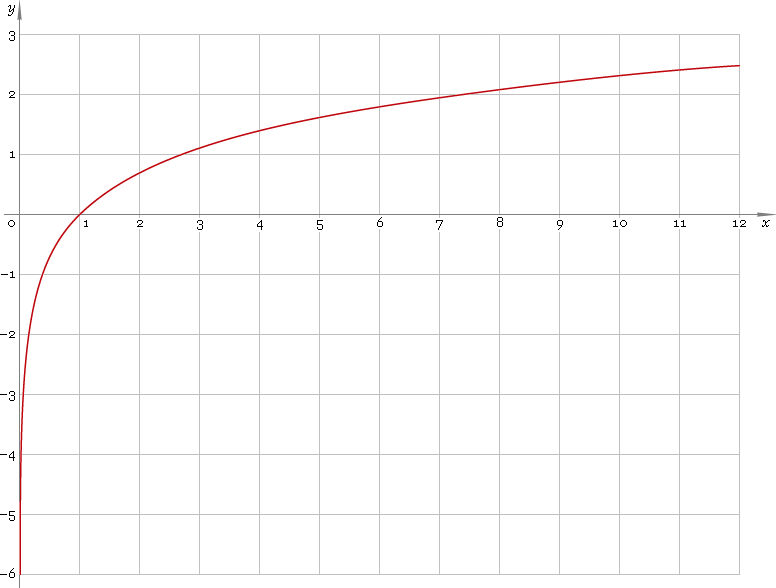The Art of Mathematics

# ln — natural logarithmic function

## 1. Definition

Natural logarithmic function is the inverse of the exponential function.

## 2. Graph

Natural logarithmic function is defined on positive part of the real axis — so its domain is (0, +∞). 0 is a singular point. Function graph is depicted below — fig. 1.Fig. 1. Graph of the natural logarithmic function y = lnx.

Function codomain is entire real axis.

## 3. Identities

By definition:

ln exx

Reciprocal argument:

ln(1/x) = −lnx

Product and ratio of arguments:

ln(xy) = lnx + lny
ln(x /y) = lnx − lny

Power of argument:

lnxa = a lnx

Base change:

logax = lnx /lna
logax = logbx /logba

## 4. Derivative and indefinite integral

Natural logarithm derivative:

ln′x = 1 /x

As well:

ln′|x| = 1 /x

Indefinite integral of the natural logarithm:

∫ lnx dx = x lnxx + C

where C is an arbitrary constant.

## 5. How to use

To calculate natural logarithm of the number:

``ln(2);``

To get natural logarithm of the complex number:

``ln(2+i);``

To get natural logarithm of the current result:

``ln(rslt);``

To get natural logarithm of the number z in calculator memory:

``ln(mem[z]);``

## 6. Support

Natural logarithm of the real argument is supported in free version of the Librow calculator.

Natural logarithm of the complex argument is supported in professional version of the Librow calculator.